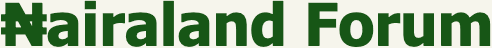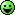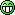#Welcome, Guest: Register On Nairaland / LOGIN! / Trending / Recent / New
Stats: 2,936,288 members, 7,105,071 topics. Date: Thursday, 06 October 2022 at 10:58 AM

## Nairaland Mathematics Clinic - Education (7) - Nairaland

 Re: Nairaland Mathematics Clinic by BrutusOj(m): 2:51pm On Jan 03, 2013 Tolzeal: Thatx the solun at ma picx..jus us what popular kald almyty 4mula..andu gewd to go..i wil pwt ma fnal anx sn.. nice one dear...I can see we have inteligent ppl on Nairaland...I actualy enjoy dis vry tread,brain exercise....thumbs up Re: Nairaland Mathematics Clinic by Odelola01(m): 2:52pm On Jan 03, 2013 State the Lami's law and parallelogram law of forces? Re: Nairaland Mathematics Clinic by ositadima1(m): 2:58pm On Jan 03, 2013 BrutusOj: Waoo..nice tread.my gurus in d house,kindly make t d subject of d formular in d expresion below" s=ut+1/2at^2" I.e d second equation of motion(s=ut+half atsquare).am waitinTo solve this one I am going to use the completing the square method... Re: Nairaland Mathematics Clinic by Mathano(m): 3:02pm On Jan 03, 2013 D parallelogram law of vectors states dat if two forces are represented by d base and height of a parallelogram den their resultant is d diagonal drawn 4rm a common point. Re: Nairaland Mathematics Clinic by Tolzeal(m): 3:03pm On Jan 03, 2013 ositadima1: This question is funny, if we assume a, b, c to be whole numbers then question is wrong. Why?a^2+b^2+c^2=16 a, b, c can either be -1, -2, -3 or 1, 2, 3 given dat 4 go be fu.ckup. I am still solving oooooo......... a, b, c have to be fractions... lemme Try Re: Nairaland Mathematics Clinic by beto2gbaski: 3:09pm On Jan 03, 2013 @OP and my great maths gurus in the house. I luv maths and even tho I never applied to study it in skul, I must say it was a rily nice and intruiging experience. My problem is dat I had some lecturers who simply had no idea wat dey were doing. For example, up till now - almost 14 months after graduating- my knowledge of PDE's and Lebesque measure/integral is a mess. Suffice to say for now dat I wud just like a quick overview of Fibonachi Sequences. Tanks and God bless. Re: Nairaland Mathematics Clinic by ositadima1(m): 3:12pm On Jan 03, 2013 BrutusOj: Waoo..nice tread.my gurus in d house,kindly make t d subject of d formular in d expresion below" s=ut+1/2at^2" I.e d second equation of motion(s=ut+half atsquare).am waitinTo solve this one I am going to use the completing the square method...s=ut+(0.5)at^2Multiply by 2/a2s/a=(2u/a)t+t^2t^2+(2u/a)t+....=2s/a+....Now, to make our equation a perfect square we add (u/a)^2 to both sides.t^2+(2u/a)t+(u/a)^2=2s/a+(u/a)^2Lets factor this baby...(t+u/a)^2=2s/a+(u/a)^2t+u/a=(2s/a+(u/a)^2)^0.5note its plus minus, meaning u have two answerst=(2s/a+(u/a)^2)^0.5-u/at=-(2s/a+(u/a)^2)^0.5-u/a 1 Like Re: Nairaland Mathematics Clinic by BrutusOj(m): 3:12pm On Jan 03, 2013 Odelola01: State the Lami's law and parallelogram law of forces? to state it mathematically since we re mor interested in mathematics.it states that" F1/sin(teta)=F2/sin(beta)=F3/sin(gama)I.e if three forces F1,F2 n F3 are in equilibruim,den d magnitude of each force is proportional to d sine of d angle btwn d other two forces.teta is d angle btw F2 n F3,beta is d angle btwn F1 n F3 n gama is d angle btwn F1 n F2.Lami' theorem is what we have as sine rule in math.Parallelogram law of vectors(forces) is d popular cosine rule or pythagoras theorem..if two forces are represented in magnitute n direction by d adjacent sides of a parallelogram,dn dia resultant is d diagonal from d comon point R=A^2+B^2-2ABcos(180-teta) where teta is d angle btwn A n B 1 Like Re: Nairaland Mathematics Clinic by BrutusOj(m): 3:13pm On Jan 03, 2013 Odelola01: State the Lami's law and parallelogram law of forces? to state it mathematically since we re mor interested in mathematics.it states that" F1/sin(teta)=F2/sin(beta)=F3/sin(gama)I.e if three forces F1,F2 n F3 are in equilibruim,den d magnitude of each force is proportional to d sine of d angle btwn d other two forces.teta is d angle btw F2 n F3,beta is d angle btwn F1 n F3 n gama is d angle btwn F1 n F2.Lami' theorem is what we have as sine rule in math.Parallelogram law of vectors(forces) is d popular cosine rule or pythagoras theorem..if two forces are represented in magnitute n direction by d adjacent sides of a parallelogram,dn dia resultant is d diagonal from d comon point R^2=A^2+B^2-2ABcos(180-teta) where teta is d angle btwn A n B Re: Nairaland Mathematics Clinic by dabrake(m): 3:25pm On Jan 03, 2013 biolabee: truman u are a demona + b + c = 8(a+b+c) ^2 = 64====> a2 + b2 + c2 + 2(ab+ac+bc) = 64====> hence ab+ac+bc = 48/2 = 24 ------------(1)(a+b+c)^3 = 512a3+b3+c3+ 3a(b2+c2+ + 3b(a2+c2) + 3c(a2+b2) + 6abc = 512I only need one more equation product of abcI don tirei continued from here, solved another 2 pages..........And I arrived at equation 1(a + b + c) = 8.E neva happen before 1 Like 1 Share Re: Nairaland Mathematics Clinic by ositadima1(m): 3:28pm On Jan 03, 2013 double post Re: Nairaland Mathematics Clinic by ositadima1(m): 3:29pm On Jan 03, 2013 dabrake: i continued from here, solved another 2 pages..........And I arrived at equation 1(a + b + c) = 8.E neva happen beforeHahahaha, what d dude did was wrong sha(a+b+c) ^2 = 64 this makes no sense Re: Nairaland Mathematics Clinic by Tolzeal(m): 3:29pm On Jan 03, 2013 dabrake: i continued from here, solved another 2 pages..........And I arrived at equation 1(a + b + c) = 8.E neva happen before it a fogd questn I think...am n ma 5th page solvn stil no ansa... Re: Nairaland Mathematics Clinic by ositadima1(m): 3:32pm On Jan 03, 2013 Tolzeal: it a fogd questn I think...am n ma 5th page solvn stil no ansa...Men, we have three variables and third power here, this ish is too complex to be solved by hand Re: Nairaland Mathematics Clinic by Tolzeal(m): 3:44pm On Jan 03, 2013 dabrake: i continued from here, solved another 2 pages..........And I arrived at equation 1(a b c) = 8.E neva happen before it a fogd questn I think...am n ma 5th page solvn stil no ansa... Re: Nairaland Mathematics Clinic by Truman155(m): 3:48pm On Jan 03, 2013 Its not a fodged question....... Its just complex. Re: Nairaland Mathematics Clinic by cigie(m): 3:52pm On Jan 03, 2013 olasesi: find the SUM of the nth term of the sequence.........4+44+444+4444+44444+444444+......it was given as an assignment for us back then in pre-degree...............only me got the answer to it .......i felt fulfilled then.................I HOPE THERE ARE OTHER PERSONS WHO CAN SOLVE IT....... Summation of (4*10^(n-1) + 4)Abi? Re: Nairaland Mathematics Clinic by awesomebenji(m): 3:55pm On Jan 03, 2013 2b sincere, dis' d most educative thread I've evr read. Guy, if u could help, I av a Postgraduate math questn whc am xpectd 2 prove. It's a simultaneous eqn with 3unknowns. I'll post it in d evening. Thanks 4 keeping warm 4d questn. Re: Nairaland Mathematics Clinic by dabrake(m): 3:58pm On Jan 03, 2013 Truman155: Its not a fodged question....... Its just complex. I think I'm getting closer to the answer Re: Nairaland Mathematics Clinic by Richiez(m): 4:05pm On Jan 03, 2013 a sysytem of equations is said to be inconsistent if it has no definite solution..... Re: Nairaland Mathematics Clinic by Proflakes: 4:19pm On Jan 03, 2013 Pls can anybody solve dis qustn for miFundamental frequency =50hz, fundamental current=960A, fundamental voltage= 1500V, calculate the 2nd to 15th harmonic current and voltage of the system. Re: Nairaland Mathematics Clinic by Proflakes: 4:24pm On Jan 03, 2013 x+cosx=2.154, find x Re: Nairaland Mathematics Clinic by Richiez(m): 4:28pm On Jan 03, 2013 i'm sorry i went off, i'm back to answer some of your questionseinstein_e: Can any mathematician here solve this simple question:You are about to play a dice game where you are paid 5 naira each time you roll an odd number. You are allowed to roll the dice five times. A friend tells you that the die used is biased such that even numbers are twice as likely to appear as odd numbers. Based on this information, what is the maximum amount you would pay to play this game?SOLUTIONlet p(even)=x and p(odd)=yfrom the question, p(even)= 2*p(odd)therefore; x=2y........(1)now be careful because the ratio p(even): p(odd)= 2 : 1summation of ratios= 2+1 =3hence, p(even)= 2/3 and p(odd) = 1/3no of time to roll dice = 5therefore no of odd number outcomes(also known as expectation) = 1/3 * 5 = 2.67 (approx)max. amt to be paid = 5naira * 2.67 =13.35 naira 1 Like Re: Nairaland Mathematics Clinic by Mathano(m): 4:34pm On Jan 03, 2013 Fibonacci was an italian mathematician,he developed d fibonacci series.d cci pronounced as shi.d series is just a series in which d nth term is gotten by d summation of d first two preceeding terms i.e T3=T1+T2.d series goes thus 1 1 2 3 5 8 13 till infinity.when writing a computer program 4 it.d first two terms are initialized as one. Re: Nairaland Mathematics Clinic by ositadima1(m): 4:46pm On Jan 03, 2013 Proflakes: x+cosx=2.154, find xx+cosx=2.154x+cosx=2.154+0.ix+[e^(-ix)]/2+[e^(ix)]/2=2.154x=3.15392 (appr.)converted cosx to its hyperbolic formlol, ok I cheated....The realistic way to solve it is to plot y=x+cosx against y=2.154, if u are good at graphs u should get 3.1 something at there intrsection, mind u cosx is in radians. Re: Nairaland Mathematics Clinic by dabrake(m): 4:46pm On Jan 03, 2013 Truman, your question eh. Re: Nairaland Mathematics Clinic by BecaciaBarbie(f): 4:53pm On Jan 03, 2013 Nice thread! I love Mathematics, but hate to solve any... Re: Nairaland Mathematics Clinic by bakenda(m): 4:58pm On Jan 03, 2013 math-diva:Thumbs up guys for the good job. I studied pure maths in unilag and I must say that my life has been the better for it. For all those who have the passion for maths I encourage you to study it in school and learn to make your passion work for you. Maths forms the base of all science and engineering courses so it's very easy to convert to other professions. A lot of my colleagues work in banks, audit firms, marketing firms, oil and gas, telecoms, food and beverages etcI remember in 300 and final year I rarely used my calculator cos most of the courses we did were theoretical and used more of x, y and z rather than numbers. Then I was so sure Prof Olaleru was making up some of the stuff he taught us. So you can imagine how surprised I was to find all those pure maths principles embedded in stuff I do at work.What am just trying to tell you all who want to know if it's worth their time to study maths or stats the answer is yes, especially if your a natural guru. All the maths gurus in my class spent more time playing games than reading, in maths once u know it you know it and unless your ecturer na witch, there is no way he wants to set his questions that u will not answer it and get method mark.So Dr. Olaleru is now a Prof., congrats to him. Re: Nairaland Mathematics Clinic by 1stknight(m): 5:11pm On Jan 03, 2013 dabrake: Truman, your question eh.Have you solved truman question? Re: Nairaland Mathematics Clinic by Nobody: 5:20pm On Jan 03, 2013 Youngsage: x + y =5 -------- eqn i.x^x + y^y=31 ----- eqn ii.From equatns i & ii, take the log of both sides(xlog^x + ylog^y) = log 31------ eqn iii.logx + logy = log 5 ------------ eqn iv. xylogxy= log 31 -------- eqn v. log xy = log 5 --------- eqn vi. (Using elimination method);xylog5 = log31xy = log 31/log 5 =6.2 approx. 6.Recall, from eqn i, x + y = 5.So x = 6/y or y = 6/x.Therefore x=2 when y=3.ans: x=2, and y=3.Log(x+y) = Log 5.. Is it the same as log x + log y = Log 5?? Cross check your solvings.. 1 Like Re: Nairaland Mathematics Clinic by dabrake(m): 5:36pm On Jan 03, 2013 1stknight: Have you solved truman question?nooooh!Though, the question isn't wrong. I proved it wasn't but to find that a, b or c na die. Hopefully, the answer will appear before the day runs out. Re: Nairaland Mathematics Clinic by ositadima1(m): 5:49pm On Jan 03, 2013 dabrake: nooooh!Though, the question isn't wrong. I proved it wasn't but to find that a, b or c na die. Hopefully, the answer will appear before the day runs out.Guy dont stress ur self, I used a programming calc. and I got this answer;a=4.87119b=1.5644-2.51229ic=1.5644+2.51229iThis is one set of answers, they are like 3 set total and they are all complex numbers, I doubt anybody can solve this equations by hand.If a+b+c=8, a^2+b^2+c^2=16 and a^3+ b^3+c^3=64 find a,b, and c 1 Like

(Go Up)

 Sections: politics (1) business autos (1) jobs (1) career education (1) romance computers phones travel sports fashion health religion celebs tv-movies music-radio literature webmasters programming techmarket Nairaland - Copyright © 2005 - 2022 Oluwaseun Osewa. All rights reserved. See How To Advertise. 124Disclaimer: Every Nairaland member is solely responsible for anything that he/she posts or uploads on Nairaland.